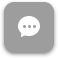Call Now 855-300-1469

# MGT 5006 Introductory Managerial Statistics

#### Course Description

Explores methods of collecting, analyzing and interpreting data for managerial decision making. Includes data presentation, measures of central tendency, dispersion, and skewness; discrete and continuous probability distributions; sampling methods and sampling distributions; and confidence interval estimation of parameters and tests of hypotheses.

#### Course Objectives

• Present data, calculate and interpret measures of central tendency and dispersion, and interpret these meanings
• Solve problems involving elementary probabilities and interpret their results
• Identify and use various types of probability distributions to solve relevant problems
• Use sampling distributions to construct confidence intervals and estimates of population parameters
• Test statistical hypotheses using the z and t distributions
• Develop and evaluate statistical applications in quality and productivity management

### Week 1

##### Lecture: Tabulating and Graphing Categorical Data

Outcomes

• Present data, calculate, and interpret measures of central tendency and dispersion, and interpret these meanings
• Solve problems involving elementary probabilities and interpret their results
• Identify and use various types of probability distributions to solve relevant problems
• Use sampling distributions to construct confidence intervals and estimates of population parameters
• Test statistical hypotheses using the z and t distributions
• Develop and evaluate statistical applications in quality and productivity management

### Week 8

##### Lecture: Integrated Quality and Process Management

The course description, objectives and learning outcomes are subject to change without notice based on enhancements made to the course. October 2013